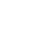DEMO 试玩

## 前言## 正题开始

### 需求分析### 技术方案概述### 核心一：图像采集与展示

#### 图像采集```navigator.mediaDevices.getUserMedia(constraints)
.then(function(stream) {
/* use the stream */
})
.catch(function(err) {
/* handle the error */
});```

```class App extends Component {
constructor(props) {
super(props);
// ...
this.stream = null;
this.video = null;
// ...
}
componentDidMount() {
// ...
this.startMedia();
}
startMedia = () => {
const constraints = {
audio: false,
video: true,
};
.then(this.handleSuccess)
.catch(this.handleError);
}
handleSuccess = (stream) => {
this.stream = stream; // 获取视频流
this.video.srcObject = stream; // 传给 video
}
handleError = (error) => {
console.log('navigator.getUserMedia error: ', error);
}
// ...
}```

#### 实时展示

`canvas.getContext('2d').drawImage(video, 0, 0, canvas.width, canvas.height);`

```export const drawBox = ({ ctx, x, y, w, h, emoji }) => {
ctx.strokeStyle = EmojiToColor[emoji];
ctx.lineWidth = '4';
ctx.strokeRect(x, y, w, h);
}
export const drawText = ({ ctx, x, y, text }) => {
ctx.fillStyle = '#ff6347'
ctx.font = '16px'
ctx.textBaseline = 'top'
}```

### 核心二：模型预测

#### 人脸提取```export class FaceExtractor {
constructor(path = MODEL_PATH, params = PARAMS) {
this.path = path;
this.params = params;
}
this.model = new faceapi.Mtcnn();
}
async findAndExtractFaces(img) {
// ...一些基本判空保证在加载好后使用
const input = await faceapi.toNetInput(img, false, true);
const results = await this.model.forward(input, this.params);
const detections = results.map(r => r.faceDetection);
const faces = await faceapi.extractFaces(input.inputs, detections);
return { detections, faces };
}
}```

#### 情绪分类```const NORM_OFFSET = tf.scalar(127.5);
export const normImg = (img, size) => {
// 转换成张量
const imgTensor = tf.fromPixels(img);
// 从 [0, 255] 标准化到 [-1, 1].
const normalized = imgTensor
.toFloat()
.sub(NORM_OFFSET) // 中心化
.div(NORM_OFFSET); // 标准化
const { shape } = imgTensor;
if (shape === size && shape === size) {
return normalized;
}
// 按照指定大小调整
const alignCorners = true;
return tf.image.resizeBilinear(normalized, [size, size], alignCorners);
}```

```export const rgbToGray = async imgTensor => {
const minTensor = imgTensor.min()
const maxTensor = imgTensor.max()
const min = (await minTensor.data())
const max = (await maxTensor.data())
minTensor.dispose()
maxTensor.dispose()
// 灰度图则需要标准化到 [0, 1]，按照像素值的区间来标准化
const normalized = imgTensor.sub(tf.scalar(min)).div(tf.scalar(max - min))
// 灰度值取 RGB 的平均值
let grayscale = normalized.mean(2)
// 扩展通道维度来获取正确的张量形状 (h, w, 1)
return grayscale.expandDims(2)
}``````class Model {
constructor({ path, imageSize, classes, isGrayscale = false }) {
this.path = path
this.imageSize = imageSize
this.classes = classes
this.isGrayscale = isGrayscale
}
// 预热一下
const inShape = this.model.inputs.shape.slice(1)
const result = tf.tidy(() => this.model.predict(tf.zeros([1, ...inShape])))
await result.data()
result.dispose()
}
async imgToInputs(img) {
// 转换成张量并 resize
let norm = await prepImg(img, this.imageSize)
// 转换成灰度图输入
norm = await rgbToGrayscale(norm)
// 这就是所说的设置 batch 为 1
return norm.reshape([1, ...norm.shape])
}
async classify(img, topK = 10) {
const inputs = await this.imgToInputs(img)
const logits = this.model.predict(inputs)
const classes = await this.getTopKClasses(logits, topK)
return classes
}
async getTopKClasses(logits, topK = 10) {
const values = await logits.data()
let predictionList = []
for (let i = 0; i < values.length; i++) {
predictionList.push({ value: values[i], index: i })
}
predictionList = predictionList
.sort((a, b) => b.value - a.value)
.slice(0, topK)
return predictionList.map(x => {
return { label: this.classes[x.index], value: x.value }
})
}
}
export default Model```

```// 示意用
const classifyResult = [0.1, 0.2, 0.25, 0.15, 0.3];``````// 略有调整
analyzeFaces = async (img) => {
// ...
const faceResults = await this.faceExtractor.findAndExtractFaces(img);
const { detections, faces } = faceResults;
// 对提取到的每一个人脸进行分类
let emotions = await Promise.all(
faces.map(async face => await this.emotionModel.classify(face))
);
// ...
}
drawDetections = () => {
const { detections, emotions } = this.state;
if (!detections.length) return;
const { width, height } = this.canvas;
const ctx = this.canvas.getContext('2d');
const detectionsResized = detections.map(d => d.forSize(width, height));
detectionsResized.forEach((det, i) => {
const { x, y } = det.box
const { emoji, name } = emotions[i].label;
drawBox({ ctx, ...det.box, emoji });
drawText({ ctx, x, y, text: emoji, name });
});
}```

### 实时性优化```handleSnapshot = async () => {
// ... 一些 canvas 准备操作
canvas.getContext('2d').drawImage(video, 0, 0, canvas.width, canvas.height);
this.drawDetections(); // 绘制 state 中维护的结果

// 利用 flag 判断是否有正在进行的模型预测
if (!this.isForwarding) {
this.isForwarding = true;
const imgSrc = await getImg(canvas.toDataURL('image/png'));
this.analyzeFaces(imgSrc);
}
const that = this;
setTimeout(() => {
that.handleSnapshot();
}, 10);
}
analyzeFaces = async (img) => {
// ...其他操作
const faceResults = await this.models.face.findAndExtractFaces(img);
const { detections, faces } = faceResults;
let emotions = await Promise.all(
faces.map(async face => await this.models.emotion.classify(face))
);
this.setState(
() => {
// 获取到新的预测值后，将 flag 置为 false，以再次进行预测
this.isForwarding = false;
}
);
}```

## 效果展示## One Comment

1.jonsnow

666